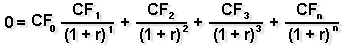Internal Rate of Return

The internal rate of return (IRR) method of analyzing a major purchase or project allows you to consider the time value of money. Essentially, it allows you to find the interest rate that is equivalent to the dollar returns you expect from your project. Once you know the rate, you can compare it to the rates you could earn by investing your money in other projects or investments.

If the internal rate of return is less than the cost of borrowing used to fund your project, the project will clearly be a money-loser. However, usually a business owner will insist that in order to be acceptable, a project must be expected to earn an IRR that is at least several percentage points higher than the cost of borrowing, to compensate the company for its risk, time, and trouble associated with the project.

As an example of how the internal rate of return works, let's say you're looking at a project costing \$7,500 that is expected to return \$2,000 per year for five years, or \$10,000 in total. The IRR calculated for the project would be 10 percent. If your cost of borrowing for the project is less than 10 percent, the project may be worthwhile. If the cost of borrowing is 10 percent or greater, it won't make sense to do the project (at least from a financial perspective) because, at best, you'll be breaking even.

IRR analysis is generally used to evaluate the project's cash flows, rather than the income from the project that would be shown on an income statement (also known as the profit and loss statement). Why? Because income on a P " L reflects depreciation, but depreciation is not an out-of-pocket expense. For instance, if revenue of \$10,000 is reduced to \$7,000 of income because of a \$3,000 depreciation deduction, you still have the use of the full \$10,000. So the cash flow figure of \$10,000 is usually the more instructive one to look at. However, if you are very concerned about the appearance of your income statement (for example, if you anticipate putting the business up for sale or seeking major financing in the future, or if you're under stockholder pressure for increased income) you may decide that the income figure is more appropriate to use.

How do you compute the IRR? The easiest way is to use a good financial calculator. If you don't have one, or don't want to take the time to learn to use one, you can also use the present value tables located in Business Tools.Among the Business Tools is a simple "present value of a series of \$1 payments" table that you can use to figure the IRR of your project.

Using the present value tables. If you want to use the present value tables to calculate the IRR of your project, you must first compute the number to look up in the tables. Do that by dividing your expected net cash outflow for the project by your expected average annual net cash inflow. For example, in our example above, the cost of the project (net cash outflow) was \$7,500, and the average annual net cash inflow was \$2,000.

 \$7,500 \$2,000 = 3.8

Then, look at the row corresponding to the number of years the project or equipment will be in use (in this case, five). Look across the rows until you find the number that is closest to the result you found (3.8). Then look at the top of the column in which the closest number was found, to see the interest rate that is your IRR (in this case, 10 percent).

One problem with the IRR is that if the expected cash inflows vary greatly from year to year, it's very difficult and time-consuming to calculate the interest rate, though it can be done by using the following math formula.

Using a math formula. For those who are mathematically inclined, you can use the following formula (which is very similar to the formula used to find the net present value. The main difference is that in the IRR formula, you must solve for the interest rate, r.(When using this formula, CFx = cash flow in period x, and n = the number of periods.)